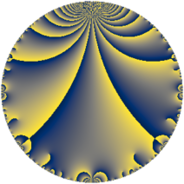# Properties

 Label 8.4.bLevel $8$ Weight $4$ Character orbit 8.b Rep. character $\chi_{8}(5,\cdot)$ Character field $\Q$ Dimension $2$ Newform subspaces $1$ Sturm bound $4$ Trace bound $0$

# Related objects

## Defining parameters

 Level: $$N$$ $$=$$ $$8 = 2^{3}$$ Weight: $$k$$ $$=$$ $$4$$ Character orbit: $$[\chi]$$ $$=$$ 8.b (of order $$2$$ and degree $$1$$) Character conductor: $$\operatorname{cond}(\chi)$$ $$=$$ $$8$$ Character field: $$\Q$$ Newform subspaces: $$1$$ Sturm bound: $$4$$ Trace bound: $$0$$

## Dimensions

The following table gives the dimensions of various subspaces of $$M_{4}(8, [\chi])$$.

Total New Old
Modular forms 4 4 0
Cusp forms 2 2 0
Eisenstein series 2 2 0

## Trace form

 $$2 q - 2 q^{2} - 12 q^{4} + 28 q^{6} - 16 q^{7} + 40 q^{8} - 2 q^{9} + O(q^{10})$$ $$2 q - 2 q^{2} - 12 q^{4} + 28 q^{6} - 16 q^{7} + 40 q^{8} - 2 q^{9} - 56 q^{10} - 56 q^{12} + 16 q^{14} + 112 q^{15} + 16 q^{16} - 28 q^{17} + 2 q^{18} + 112 q^{20} - 84 q^{22} - 304 q^{23} - 112 q^{24} + 26 q^{25} + 280 q^{26} + 96 q^{28} - 112 q^{30} + 448 q^{31} - 352 q^{32} + 168 q^{33} + 28 q^{34} + 12 q^{36} - 196 q^{38} - 560 q^{39} + 224 q^{40} - 140 q^{41} - 224 q^{42} + 168 q^{44} + 304 q^{46} + 672 q^{47} + 672 q^{48} - 558 q^{49} - 26 q^{50} - 560 q^{52} + 728 q^{54} - 336 q^{55} - 320 q^{56} + 392 q^{57} - 840 q^{58} - 672 q^{60} - 448 q^{62} + 16 q^{63} + 576 q^{64} + 1120 q^{65} - 168 q^{66} + 168 q^{68} + 448 q^{70} - 144 q^{71} - 40 q^{72} - 588 q^{73} + 1288 q^{74} + 392 q^{76} + 560 q^{78} - 928 q^{79} - 1344 q^{80} - 1510 q^{81} + 140 q^{82} + 448 q^{84} - 2324 q^{86} + 1680 q^{87} + 336 q^{88} + 532 q^{89} + 56 q^{90} + 1824 q^{92} - 672 q^{94} - 784 q^{95} - 448 q^{96} + 1988 q^{97} + 558 q^{98} + O(q^{100})$$

## Decomposition of $$S_{4}^{\mathrm{new}}(8, [\chi])$$ into newform subspaces

Label Dim $A$ Field CM Traces $q$-expansion
$a_{2}$ $a_{3}$ $a_{5}$ $a_{7}$
8.4.b.a $2$ $0.472$ $$\Q(\sqrt{-7})$$ None $$-2$$ $$0$$ $$0$$ $$-16$$ $$q+(-1-\beta )q^{2}+2\beta q^{3}+(-6+2\beta )q^{4}+\cdots$$